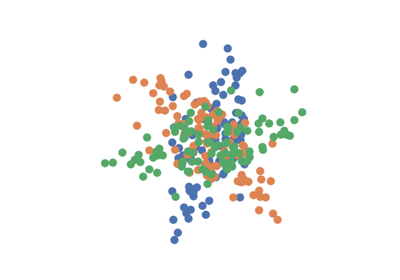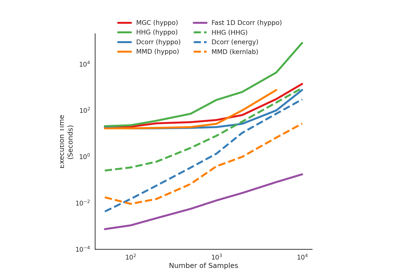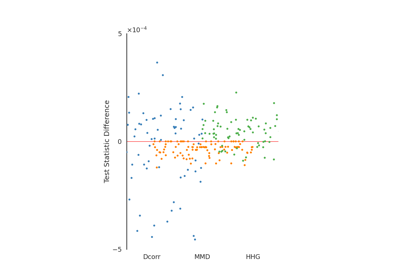# MMD¶

class hyppo.ksample.MMD(compute_kernel='gaussian', bias=False, **kwargs)

Maximum Mean Discrepency (MMD) test statistic and p-value.

MMD is a powerful multivariate 2-sample test. It leverages kernel similarity matrices capabilities (similar to tests like distance correlation or Dcorr). In fact, MMD statistic is equivalent to our 2-sample formulation nonparametric MANOVA via independence testing, i.e. hyppo.ksample.KSample, and to hyppo.independence.Dcorr, hyppo.ksample.DISCO, hyppo.independence.Hsic, and hyppo.ksample.Energy 1 2.

Parameters
• compute_kernel (str, callable, or None, default: "gaussian") -- A function that computes the kernel similarity among the samples within each data matrix. Valid strings for compute_kernel are, as defined in sklearn.metrics.pairwise.pairwise_kernels,

["additive_chi2", "chi2", "linear", "poly", "polynomial", "rbf", "laplacian", "sigmoid", "cosine"]

Note "rbf" and "gaussian" are the same metric. Set to None or "precomputed" if x and y are already similarity matrices. To call a custom function, either create the similarity matrix before-hand or create a function of the form metric(x, **kwargs) where x is the data matrix for which pairwise kernel similarity matrices are calculated and kwargs are extra arguements to send to your custom function.

• bias (bool, default: False) -- Whether or not to use the biased or unbiased test statistics.

• **kwargs -- Arbitrary keyword arguments for compute_kernel.

Notes

Traditionally, the formulation for the 2-sample MMD statistic is as follows 3:

Define $$\{ u_i \stackrel{iid}{\sim} F_U,\ i = 1, ..., n \}$$ and $$\{ v_j \stackrel{iid}{\sim} F_V,\ j = 1, ..., m \}$$ as two groups of samples deriving from different distributions with the same dimensionality. If $$k(\cdot, \cdot)$$ is a kernel metric (i.e. Gaussian) then,

$\mathrm{MMD}_{n, m}(\mathbf{u}, \mathbf{v}) = \frac{1}{m(m - 1)} \sum_{i = 1}^m \sum_{j \neq i}^m k(u_i, u_j) + \frac{1}{n(n - 1)} \sum_{i = 1}^n \sum_{j \neq i}^n k(v_i, v_j) - \frac{2}{mn} \sum_{i = 1}^n \sum_{j \neq i}^n k(v_i, v_j)$

The implementation in the hyppo.ksample.KSample class (using hyppo.independence.Hsic using 2 samples) is in fact equivalent to this implementation (for p-values) and statistics are equivalent up to a scaling factor 2.

The p-value returned is calculated using a permutation test uses hyppo.tools.perm_test. The fast version of the test uses hyppo.tools.chi2_approx.

References

1

Sambit Panda, Cencheng Shen, Ronan Perry, Jelle Zorn, Antoine Lutz, Carey E. Priebe, and Joshua T. Vogelstein. Nonpar MANOVA via Independence Testing. arXiv:1910.08883 [cs, stat], April 2021. arXiv:1910.08883.

2(1,2)

Cencheng Shen and Joshua T. Vogelstein. The exact equivalence of distance and kernel methods in hypothesis testing. AStA Advances in Statistical Analysis, September 2020. doi:10.1007/s10182-020-00378-1.

3

Arthur Gretton, Karsten M. Borgwardt, Malte J. Rasch, Bernhard Schölkopf, and Alexander Smola. A Kernel Two-Sample Test. Journal of Machine Learning Research, 13(25):723–773, 2012.

Methods Summary

 MMD.statistic(x, y) Calulates the MMD test statistic. MMD.test(x, y[, reps, workers, auto, ...]) Calculates the MMD test statistic and p-value.

MMD.statistic(x, y)

Calulates the MMD test statistic.

Parameters

x,y (ndarray) -- Input data matrices. x and y must have the same number of dimensions. That is, the shapes must be (n, p) and (m, p) where n is the number of samples and p and q are the number of dimensions.

Returns

stat (float) -- The computed MMD statistic.

MMD.test(x, y, reps=1000, workers=1, auto=True, random_state=None)

Calculates the MMD test statistic and p-value.

Parameters
• x,y (ndarray) -- Input data matrices. x and y must have the same number of dimensions. That is, the shapes must be (n, p) and (m, p) where n is the number of samples and p and q are the number of dimensions.

• reps (int, default: 1000) -- The number of replications used to estimate the null distribution when using the permutation test used to calculate the p-value.

• workers (int, default: 1) -- The number of cores to parallelize the p-value computation over. Supply -1 to use all cores available to the Process.

• auto (bool, default: True) -- Automatically uses fast approximation when n and size of array is greater than 20. If True, and sample size is greater than 20, then hyppo.tools.chi2_approx will be run. Parameters reps and workers are irrelevant in this case. Otherwise, hyppo.tools.perm_test will be run.

Returns

Examples

>>> import numpy as np
>>> from hyppo.ksample import MMD
>>> x = np.arange(7)
>>> y = x
>>> stat, pvalue = MMD().test(x, y)
>>> '%.3f, %.1f' % (stat, pvalue)
'-0.015, 1.0'


## Examples using hyppo.ksample.MMD¶